• ## 图像去模糊（一）——理解模糊核

万次阅读 多人点赞 2016-10-10 23:30:02
视频图像在采集生成、传输、转换、接收和处理的过程中，由于各种原因不可避免地会导致不同程度的视频图像质量的下降，这过程被称为视频图像的退化。运动视频图像去模糊...本文主要讲述去模糊过程中模糊核的理解。
1、数学模型 　　当用相机拍摄物体时，物体运动、相机抖动或者物体失焦会造成图像模糊，降低图像的视觉质量。这个过程即为图像退化。图像模糊一般被看作清晰图像卷积模糊核得到模糊图像的过程，其退化模型如下式所示：其中，B为模糊图像( blurry image )，I为待估计的清晰图像( latent image )，K为模糊核( blur kernel )，N为附加的噪声，ⓧ为卷积操作。
2、图像卷积基本概念
2.1 线性滤波 　　线性滤波是图像处理的基本方法。假设我们有图像矩阵A和滤波器矩阵（卷积核）B，如下图所示：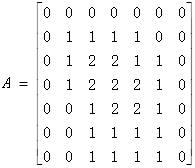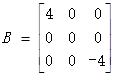对于图像的每一个像素点，计算它的邻域像素和滤波器矩阵的对应元素的乘积，然后加起来，作为该像素位置的值。这样就完成了滤波过程。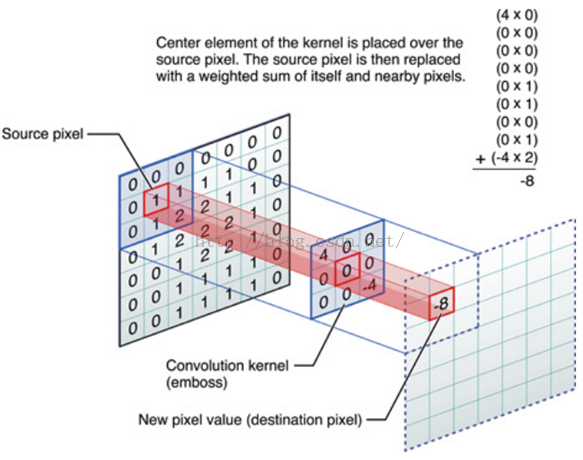2.2 卷积与相关 　　对图像和滤波矩阵进行逐个元素相乘再求和的操作就相当于将一个二维的函数移动到另一个二维函数的所有位置，这个操作就叫卷积或者相关。卷积和相关的差别是，卷积需要先对滤波矩阵进行180的翻转，但如果矩阵是对称的，那么两者就没有什么差别了。
3、模糊核（卷积核） 　　模糊核实际上就是一个矩阵，清晰图像与模糊核卷积后导致图像变得模糊，因此叫模糊核。模糊核是卷积核的一种。图像卷积操作的本质是矩阵卷积。某些特殊的卷积核会使图像产生特殊的效果。
3.1 边缘检测 1）Sobel算子 横向边缘检测：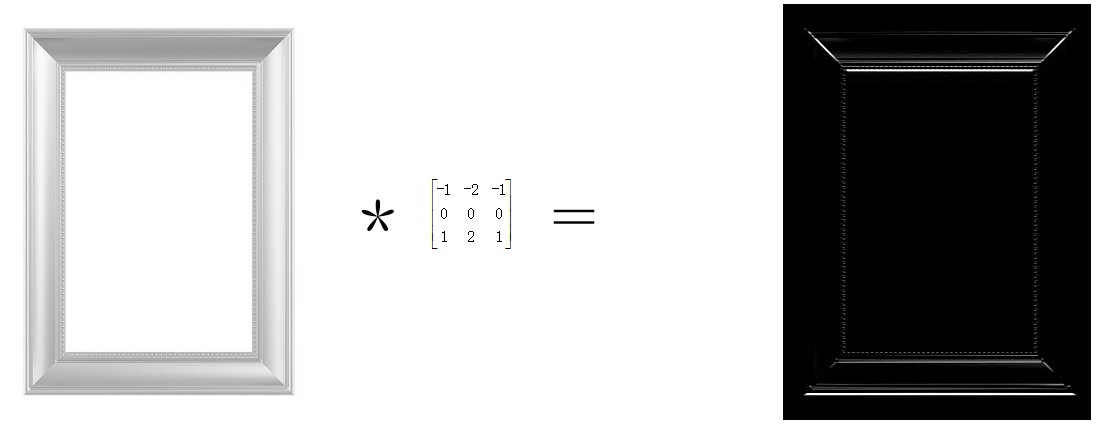纵向边缘检测：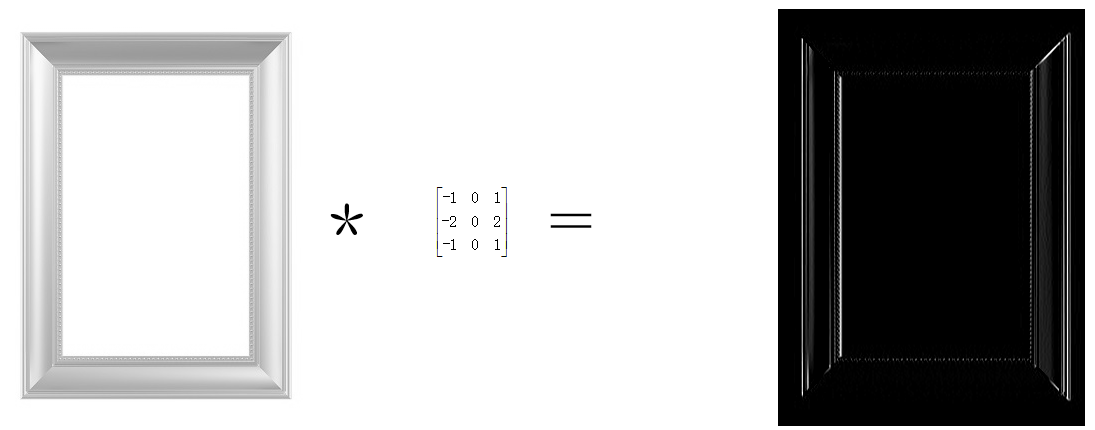2）Prewitt算子 横向边缘检测算子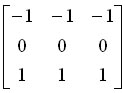和纵向边缘检测算子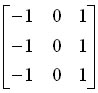。其效果与Sobel算子几乎一致，但Prewitt算子对噪声相对不敏感。
3）Laplacian算子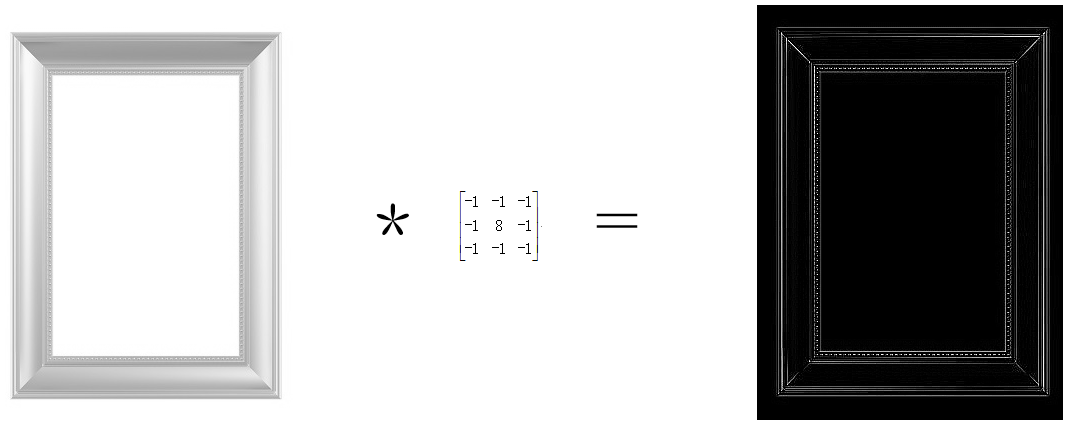3.2 图像锐化 　　图像的锐化和边缘检测很像，首先找到边缘，然后把边缘加到原来的图像上面，这样就强化了图像的边缘，使图像看起来更加锐利了。这两者操作统一起来就是锐化滤波器了，也就是在边缘检测滤波器的基础上，再在中心的位置加1，这样滤波后的图像就会和原始的图像具有同样的亮度了，但是会更加锐利。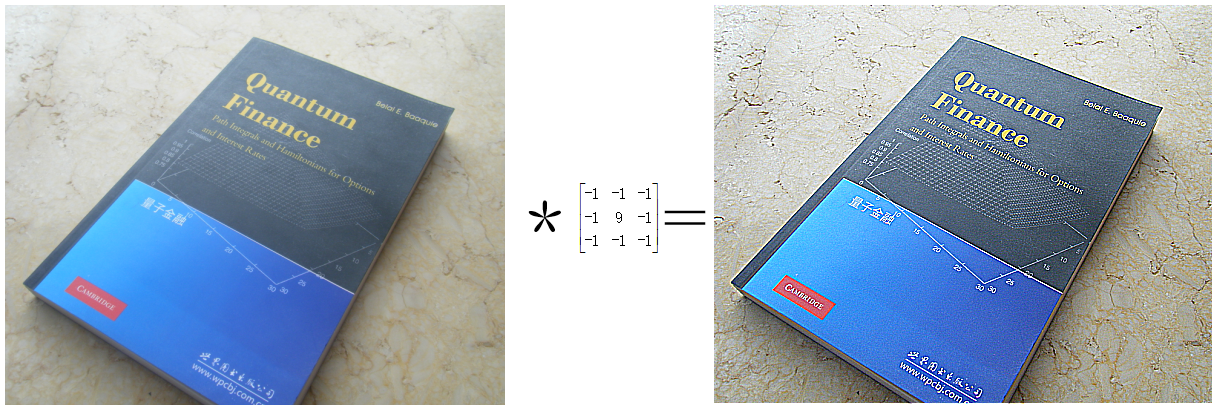下面的卷积核更强调边缘：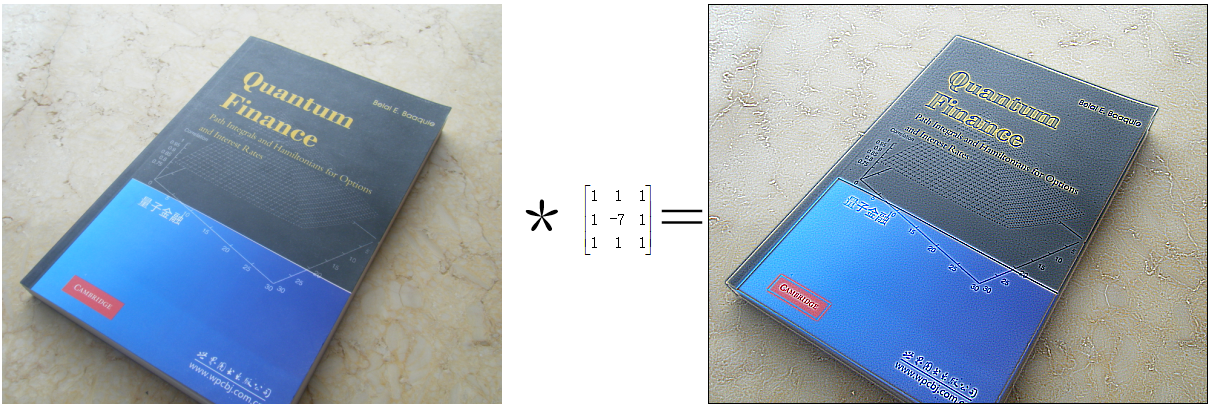3.3 浮雕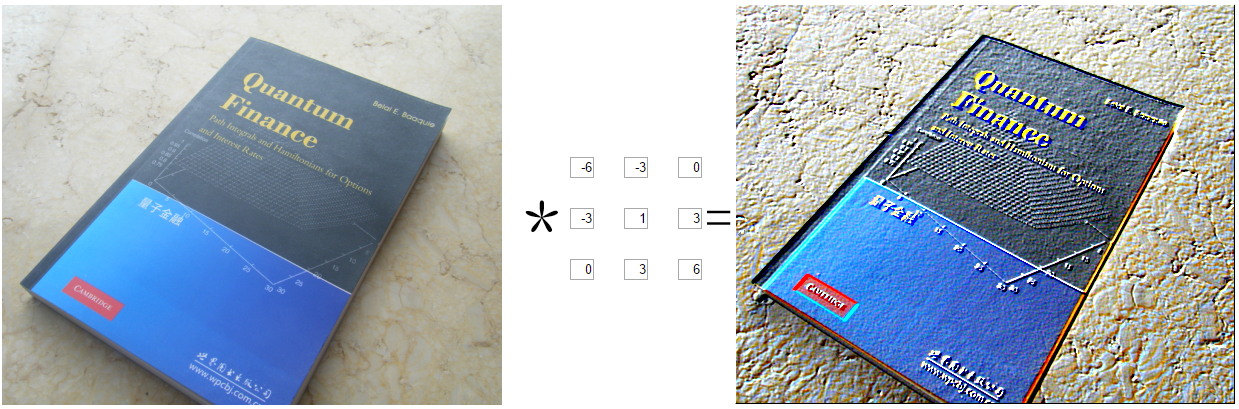3.4 运动模糊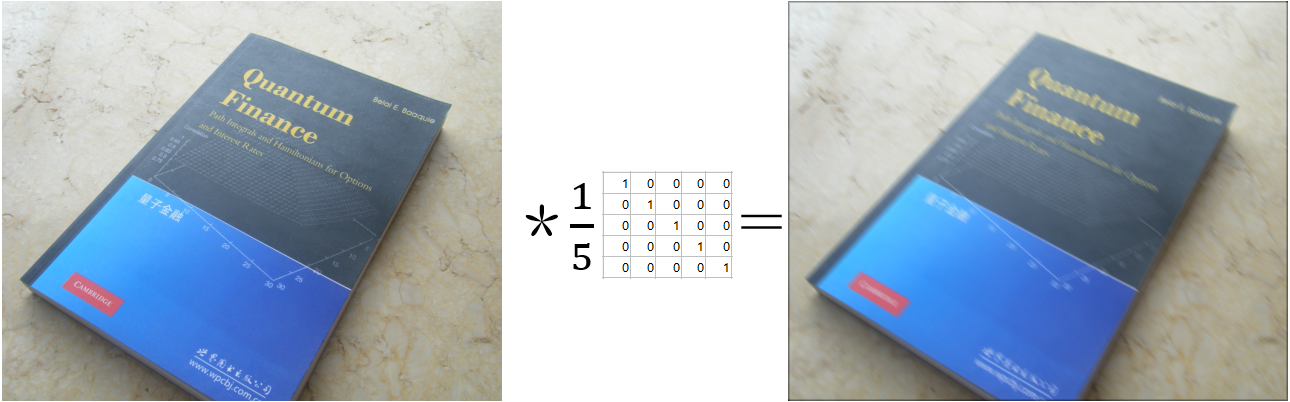展开全文kernel 图像卷积
• 数学基础: 二维高斯模糊的数学表达如下： 简单表达为G(x, y) = G(x)G(y)由此，对一个二维的像素矩阵可以分别在水平与垂直...生成一维高斯的代码如下，其中对高斯实现归一化预处理 private float[] generat
数学基础:
二维高斯模糊的数学表达如下：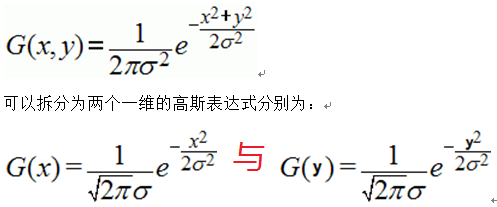简单表达为G(x, y) = G(x)G(y)由此，对一个二维的像素矩阵可以分别在水平与垂直方向
进行一维高斯模糊其效果等同与二维高斯模糊效果。由于计算量的减少，速度却比二维
高斯模糊更快。
运行结果：关键代码解析：
生成一维高斯核的代码如下，其中对高斯核实现归一化预处理

private float[] generateGaussianKeneral(int n, float sigma) {
float sigma22 = 2*sigma*sigma;
float Pi2 = 2*(float)Math.PI;
float sqrtSigmaPi2 = (float)Math.sqrt(Pi2) * sigma ;
int size = 2*n + 1;
int index = 0;
gaussianKeneral = new float[size];
float sum = 0.0f;
for(int i=-n; i<=n; i++) {
float distance = i*i;
gaussianKeneral[index] = (float)Math.exp((-distance)/sigma22)/sqrtSigmaPi2;
//System.out.println("\t" + gaussianKeneral[index]);
sum += gaussianKeneral[index];
index++;
}

// nomalization to 1
for(int i=0; i<gaussianKeneral.length; i++) {
gaussianKeneral[i] = gaussianKeneral[i]/sum;
//System.out.println("final gaussian data " + i + " = " + gaussianKeneral[i]);
}
return gaussianKeneral;
}
实现对越界像素值的处理：

public static int clamp(float a) {
return (int)(a < 0 ?  0 : ((a > 255) ? 255 : a));
}
全部算法源代码如下：

package com.gloomyfish.filter.study;

import java.awt.image.BufferedImage;

public class HVGaussianFilter extends AbstractBufferedImageOp {
private float[] gaussianKeneral = null;
private int radius = 2; // default value
private float sigma = 10;
public HVGaussianFilter() {

}

public void setSigma(float a) {
this.sigma = a;
}

}

public float[][] getHVGaussianKeneral() {
float[][] hvKeneralData = new float;
for(int i=0; i<5; i++)
{
for(int j=0; j<5; j++)
{
hvKeneralData[i][j] = gaussianKeneral[i] * gaussianKeneral[j];
}
}
return hvKeneralData;
}

@Override
public BufferedImage filter(BufferedImage src, BufferedImage dest) {
int width = src.getWidth();
int height = src.getHeight();

if ( dest == null )
dest = createCompatibleDestImage( src, null );

int[] inPixels = new int[width*height];
int[] outPixels = new int[width*height];
getRGB( src, 0, 0, width, height, inPixels);
blur( inPixels, outPixels, width, height); // H Gaussian
blur( outPixels, inPixels, height, width); // V Gaussain
setRGB(dest, 0, 0, width, height, inPixels );
return dest;
}

/**
* <p> here is 1D Gaussian        , </p>
*
* @param inPixels
* @param outPixels
* @param width
* @param height
*/
private void blur(int[] inPixels, int[] outPixels, int width, int height)
{
int subCol = 0;
int index = 0, index2 = 0;
float redSum=0, greenSum=0, blueSum=0;
for(int row=0; row<height; row++) {
int ta = 0, tr = 0, tg = 0, tb = 0;
index = row;
for(int col=0; col<width; col++) {
// index = row * width + col;
redSum=0;
greenSum=0;
blueSum=0;
for(int m=-2; m<=2; m++) {
subCol = col + m;
if(subCol < 0 || subCol >= width) {
subCol = 0;
}
index2 = row * width + subCol;
ta = (inPixels[index2] >> 24) & 0xff;
tr = (inPixels[index2] >> 16) & 0xff;
tg = (inPixels[index2] >> 8) & 0xff;
tb = inPixels[index2] & 0xff;
redSum += (tr * gaussianKeneral[m + 2]);
greenSum += (tg * gaussianKeneral[m + 2]);
blueSum += (tb * gaussianKeneral[m + 2]);
}
outPixels[index] = (ta << 24) | (clamp(redSum) << 16) | (clamp(greenSum) << 8) | clamp(blueSum);
index += height;// correct index at here!!!, out put pixels matrix,
}
}
}

public static int clamp(float a) {
return (int)(a < 0 ?  0 : ((a > 255) ? 255 : a));
}

private float[] generateGaussianKeneral(int n, float sigma) {
float sigma22 = 2*sigma*sigma;
float Pi2 = 2*(float)Math.PI;
float sqrtSigmaPi2 = (float)Math.sqrt(Pi2) * sigma ;
int size = 2*n + 1;
int index = 0;
gaussianKeneral = new float[size];
float sum = 0.0f;
for(int i=-n; i<=n; i++) {
float distance = i*i;
gaussianKeneral[index] = (float)Math.exp((-distance)/sigma22)/sqrtSigmaPi2;
//System.out.println("\t" + gaussianKeneral[index]);
sum += gaussianKeneral[index];
index++;
}

// nomalization to 1
for(int i=0; i<gaussianKeneral.length; i++) {
gaussianKeneral[i] = gaussianKeneral[i]/sum;
//System.out.println("final gaussian data " + i + " = " + gaussianKeneral[i]);
}
return gaussianKeneral;
}

}

转载文章请务必注明！

展开全文• 高斯模糊，或者说高斯滤波，是图像处理中非常常见的操作。操作的核心是使用个从高斯分布中采样得到的掩膜，或者叫，和输入图片中的每个像素及其邻域进行计算，结果保存到输出图片中...
高斯模糊原理
基本概念
二维高斯模糊，或者说高斯滤波，是图像处理中非常常见的操作。操作的核心是使用一个从高斯分布中采样得到的掩膜，或者叫核，和输入图片中的每个像素及其邻域进行计算，结果保存到输出图片中。假设高斯核窗口尺寸为

(2w+1)×(2w+1)
$(2w + 1) \times (2w + 1)$，高斯分布的标准差为

σ
$\sigma$，则高斯核可以表示为矩阵的形式

G=⎡⎣⎢⎢⎢⎢⎢⎢⎢⎢G(−w,−w)⋮G(0,−w)⋮G(w,−w)………G(−w,0)⋮G(0,0)⋮G(w,0)………G(−w,w)⋮G(0,w)⋮G(w,w)⎤⎦⎥⎥⎥⎥⎥⎥⎥⎥

\mathbf G =
\begin{bmatrix}
G(-w, -w) &\dots &G(-w, 0) &\dots &G(-w, w)\\
\vdots &{} &\vdots &{} &\vdots\\
G(0, -w) &\dots &G(0, 0) &\dots &G(0, w)\\
\vdots &{} &\vdots &{} &\vdots\\
G(w, -w) &\dots &G(w, 0) &\dots &G(w, w)
\end{bmatrix}
其中

G(u,v)=1Sexp(−u22σ2−v22σ2)

G(u,v) = \dfrac{1}{S} \exp\left(-\dfrac{u^2}{2\sigma^2}-\dfrac{v^2}{2\sigma^2}\right) 其中

u
$u$ 表示行，v$v$ 表示列，

u,v∈{−w,−w+1,…,w−1,w}
$u, v \in \{-w, -w + 1, \dots, w - 1, w\}$，

S
$S$ 是归一化常数
S=∑u=−ww∑v=−wwexp(−u22σ2−v22σ2) S = \sum_{u = -w}^{w}\sum_{v = -w}^{w} \exp\left(-\dfrac{u^2}{2\sigma^2}-\dfrac{v^2}{2\sigma^2}\right) 由于高斯分布的概率密度函数的非零值区间主要集中在

(−3σ,3σ)
$(-3\sigma, 3\sigma)$ 内，所以为了保证选取的高斯核的完整性，一般取

w≈3σ
$w \approx 3\sigma$。

说完了高斯核，该说高斯模糊的表达式了。设输入图片为

X
$\mathbf X$，输出图片为

Y
$\mathbf Y$，第

i
$i$ 行第 j$j$ 列的数据表示为

X(i,j)
$X(i, j)$ 和

Y(i,j)
$Y(i,j)$，则使用窗口大小为

(2w+1)×(2w+1)
$(2w + 1)\times(2w + 1)$，标准差为

σ
$\sigma$ 的高斯核计算后的结果为

Y(i,j)=∑u=−ww∑v=−wwX(i+u,j+v)G(u,v)(1)

Y(i,j) = \sum_{u = -w}^{w} \sum_{v = -w}^{w}X(i+u, j+v)G(u, v) \tag{1}\label{labelGaussOrigExpr} 根据这个表达式，为了得到位置

(i,j)
$(i, j)$ 上的输出，需要将高斯核的中心置于输入图片的位置

(i,j)
$(i, j)$ 处，让高斯核的每一个值和输入图片对应位置上的值相乘，进行

(2w+1)×(2w+1)
$(2w + 1)\times(2w + 1)$ 次乘法计算，然后再进行

(2w+1)×(2w+1)−1
$(2w + 1)\times(2w + 1) - 1$ 次加法计算，所以时间复杂度是

O(w2)
$O(w^2)$ 的。

可分离核形式实现
但是，注意到，高斯核的表达式是可分离的。下面为了表示方便，令

g(x)=exp(−x22σ2)

g(x) = \exp\left(-\dfrac{x^2}{2\sigma^2}\right) 则有

G(u,v)=1Sg(u)g(v)

G(u, v) = \dfrac{1}{S}g(u)g(v) 那么高斯核矩阵又可以改写成归一化常数乘以一个行向量乘以一个列向量的形式，如下

G=1S⎡⎣⎢⎢⎢⎢⎢⎢⎢⎢g(−w)g(−w)⋮g(0)g(−w)⋮g(w)g(−w)… … …g(−w)g(0)⋮g(0)g(0)⋮g(w)g(0)… … …g(−w)g(w)⋮g(0)g(w)⋮g(w)g(w)⎤⎦⎥⎥⎥⎥⎥⎥⎥⎥=1S⎡⎣⎢⎢⎢⎢⎢⎢⎢⎢g(−w)⋮g(0)⋮g(w)⎤⎦⎥⎥⎥⎥⎥⎥⎥⎥×[g(−w)…g(0)…g(w)]

\begin{align}
\mathbf G &= \dfrac{1}{S} \begin{bmatrix}
g(-w)g(-w) &\dots &g(-w)g(0) &\dots &g(-w)g(w) \\
\vdots &\ &\vdots &\ &\vdots \\
g(0)g(-w) &\dots &g(0)g(0) &\dots &g(0)g(w)\\
\vdots &\ &\vdots &\ &\vdots \\
g(w)g(-w) &\dots &g(w)g(0) &\dots &g(w)g(w)
\end{bmatrix}\\
&=\dfrac{1}{S}
\begin{bmatrix}
g(-w)\\ \vdots \\ g(0) \\ \vdots \\ g(w)
\end{bmatrix}
\times
\begin{bmatrix}
g(-w) \dots  g(0) \dots g(w)
\end{bmatrix}
\end{align}
另外

S=∑u=−ww∑v=−wwg(u)g(v)=∑u=−ww[∑v=−wwg(v)]g(u)=[∑u=−wwg(u)]×[∑v=−wwg(v)]=S′×S′

\begin{align}
S &= \sum_{u = -w}^{w}\sum_{v = -w}^{w}g(u)g(v)
=\sum_{u = -w}^{w}\left[\sum_{v = -w}^{w}g(v)\right]g(u) \\
&=\left[\sum_{u = -w}^{w}g(u)\right] \times
\left[\sum_{v = -w}^{w}g(v)\right]
= S' \times S'
\end{align}
其中

S′
$S'$ 可以认为是一维高斯核的归一化系数的倒数。所以有

G=G1×G2

\mathbf G = \mathbf G_1 \times \mathbf G_2其中

G1G2=1S′[g(−w)…g(0)…g(w)]T=1S′[g(−w)…g(0)…g(w)]

\begin{align}
\mathbf G_1 &= \dfrac{1}{S'}\left[g(-w) \dots g(0) \dots g(w)\right]^{\mathrm T}\\
\mathbf G_2 &= \dfrac{1}{S'}\left[g(-w) \dots g(0) \dots g(w)\right]
\end{align} 由此可见，

G
$\mathbf G$ 可以分离成两个向量的乘积的形式。

下面对

(1)
$\eqref{labelGaussOrigExpr}$ 式进行改写

Y(i,j)=∑u=−ww∑v=−wwX(i+u,j+v)G(u,v)=∑u=−ww∑v=−wwX(i+u,j+v)1Sg(u)g(v)=∑u=−ww∑v=−wwX(i+u,j+v)1S′g(u)1S′g(v)=∑u=−ww[∑v=−wwX(i+u,j+v)1S′g(v)]1S′g(u)=∑u=−wwZ(i+u)1S′g(u)

\begin{align}
Y(i,j) &= \sum_{u = -w}^{w} \sum_{v = -w}^{w}X(i+u, j+v)G(u, v) \\
&=\sum_{u = -w}^{w} \sum_{v = -w}^{w}X(i+u, j+v)\dfrac{1}{S} g(u)g(v)\\
&=\sum_{u = -w}^{w} \sum_{v = -w}^{w}X(i+u, j+v)\dfrac{1}{S'}g(u)\dfrac{1}{S'}g(v)\\
&= \sum_{u = -w}^{w}\left[ \sum_{v = -w}^{w}X(i+u, j+v)\dfrac{1}{S'}g(v)\right]\dfrac{1}{S'}g(u)\\
&= \sum_{u = -w}^{w} Z(i + u)\dfrac{1}{S'} g(u)
\end{align}
上面的式子表明，为了获得最终的高斯滤波的结果，可以先用横向一维高斯核

G2
$\mathbf G_2$ 与输入图片

X
$\mathbf X$ 进行计算，得到中间结果

Z
$\mathbf Z$。再用纵向一维高斯核

G1
$\mathbf G_1$ 与中间结果

Z
$\mathbf Z$ 进行计算，得到输出

Y
$\mathbf Y$。利用

X(i+u,j−w),…,X(i+u,j),…,X(i+u,j+w)

X(i + u, j - w), \dots, X(i + u, j), \dots, X(i + u, j + w) 计算得到

Z(i+u,j)
$Z(i + u, j)$ 需要进行

(2w+1)
$(2w + 1)$ 次乘法计算和

2w
$2w$ 次加法计算。利用

Z(i−w,j),…,Z(i,j),…,Z(i+w,j)

Z(i - w, j), \dots, Z(i, j), \dots, Z(i + w, j) 计算得到

Y(i,j)
$Y(i, j)$ 需要进行

(2w+1)
$(2w + 1)$ 次乘法计算和

2w
$2w$ 次加法计算。总的来说，计算出

Y(i,j)
$Y(i, j)$ 的值需要进行

(4w+2)
$(4w + 2)$ 次乘法计算和

4w
$4w$ 次加法计算，时间复杂度仅为

O(w)
$O(w)$，比直接采用

(1)
$\eqref{labelGaussOrigExpr}$ 式的计算方法快了很多。但是该算法需要使用和输入图片尺寸一致的内存保存中间结果。

实例分析
基本实现
假设我们有如下

8×8
$8 \times 8$ 的数据

X=⎡⎣⎢⎢⎢⎢⎢⎢⎢⎢⎢⎢⎢⎢⎢⎢0816243240485619172533414957210182634425058311192735435159412202836445260513212937455361614223038465462715233139475563⎤⎦⎥⎥⎥⎥⎥⎥⎥⎥⎥⎥⎥⎥⎥⎥

\mathbf X =
\begin{bmatrix}
0 &1 &2 &3 &4 &5 &6 &7 \\
8 &9 &10 &11 &12 &13 &14 &15\\
16 &17 &18 &19 &20 &21 &22 &23\\
24 &25 &26 &27 &28 &29 &30 &31 \\
32 &33 &34 &35 &36 &37 &38 &39 \\
40 &41 &42 &43 &44 &45 &46 &47 \\
48 &49 &50 &51 &52 &53 &54 &55 \\
56 &57 &58 &59 &60 &61 &62 &63
\end{bmatrix}

我们要对这些数据进行窗口大小为

5×5
$5 \times 5$，标准差为

σ=1.0
$\sigma = 1.0$ 的高斯模糊。这时我们先计算纵向高斯核

G1
$\mathbf G_1$ 和横向高斯核

G2
$\mathbf G_2$ 的数值，得到

G1G2=[0.0540.2440.4030.2440.054]T=[0.0540.2440.4030.2440.054]

\begin{align}
\end{align}
下面我们用

G2
$\mathbf G_2$ 对

X
$\mathbf X$ 的每一行进行模糊计算。以计算第 0 行为例。由于高斯核的长度等于 5，所以计算前要对第 0 行的数据进行扩展，这里采用镜像对称的方式进行扩展，扩展之后的数据为

[2−1−012345676−5−]

Z
$\mathbf Z$ 的第 0 行

20.05410.2440.05400.4030.2440.0540.70610.2440.4030.2441.10920.0540.2440.4032.00030.0540.2443.00040.054…0.054↓4.00050.2440.0545.00060.4030.2445.89170.2440.4036.29460.0540.24450.054

\begin{matrix}
2 &1 &0 &1 &2 &3 &4 &5 &6 &7 &6 &5 \\
0.054 &0.244 &0.403 &0.244 &0.054\\
{} &0.054 &0.244 &0.403 &0.244 &0.054\\
{} &{} &0.054 &0.244 &0.403 &0.244 &0.054\\
{} &{} &{} &{} &{} &{} &{}\dots\\
{} &{} &{} &{} &{} &{} &0.054 &0.244 &0.403 &0.244 &0.054\\
{} &{} &{} &{} &{} &{} &{} &0.054 &0.244 &0.403 &0.244 &0.054\\
{} &{} &{} &{} &{} &{} &{}\downarrow\\
{} &{} &0.706 &1.109 &2.000 &3.000 &4.000 &5.000 &5.891 &6.294
\end{matrix}
对

X
$\mathbf X$ 的每一行都这么处理，就可以得到中间结果

Z=⎡⎣⎢⎢⎢⎢⎢⎢⎢⎢⎢⎢⎢⎢⎢⎢0.7068.70616.70624.70632.70640.70648.70656.7061.1099.10917.10925.10933.10941.10949.10957.1092.00010.00018.00026.00034.00042.00050.00058.0003.00011.00019.00027.00035.00043.00051.00059.0004.00012.00020.00028.00036.00044.00052.00060.0005.00013.00021.00029.00037.00045.00053.00061.0005.89113.89121.89129.89137.89145.89153.89161.8916.29414.29422.29430.29438.29446.29454.29462.294⎤⎦⎥⎥⎥⎥⎥⎥⎥⎥⎥⎥⎥⎥⎥⎥

\mathbf Z =
\begin{bmatrix}
0.706   &1.109   &2.000   &3.000   &4.000   &5.000   &5.891   &6.294\\
8.706   &9.109  &10.000  &11.000  &12.000  &13.000  &13.891  &14.294\\
16.706  &17.109  &18.000  &19.000  &20.000  &21.000  &21.891  &22.294\\
24.706  &25.109  &26.000  &27.000  &28.000  &29.000  &29.891  &30.294\\
32.706  &33.109  &34.000  &35.000  &36.000  &37.000  &37.891  &38.294\\
40.706  &41.109  &42.000  &43.000  &44.000  &45.000  &45.891  &46.294\\
48.706  &49.109  &50.000  &51.000  &52.000  &53.000  &53.891  &54.294\\
56.706  &57.109  &58.000  &59.000  &60.000  &61.000  &61.891  &62.294
\end{bmatrix}

下面我们用

G1
$\mathbf G_1$ 对

Z
$\mathbf Z$ 的每一列进行模糊计算。以计算第 0 列为例。第 0 列采用镜像对称的方式进行扩展，扩展之后的数据为

⎡⎣⎢⎢⎢⎢⎢⎢⎢⎢⎢⎢⎢⎢⎢⎢⎢⎢⎢⎢⎢⎢⎢⎢⎢⎢⎢16.706−−−−−8.706−−−−−0.7068.70616.70624.70632.70640.70648.70656.70648.706−−−−−40.706−−−−−⎤⎦⎥⎥⎥⎥⎥⎥⎥⎥⎥⎥⎥⎥⎥⎥⎥⎥⎥⎥⎥⎥⎥⎥⎥⎥⎥

\begin{bmatrix}
\underline{16.706}\\
\underline{8.706}\\
0.706\\
8.706\\
16.706\\
24.706\\
32.706\\
40.706\\
48.706\\
56.706\\
\underline{48.706}\\
\underline{40.706}
\end{bmatrix}
其中加了下划线的数据就是扩展的数据。下图展示了高斯核从上向下移动并计算的过程，第一个向右的箭头右边表示的是浮点数计算结果，第二个向右箭头的右边表示四舍五入后成为整数的计算结果

16.7068.7060.7068.70616.70624.70632.70640.70648.70656.70648.70640.7060.0540.2440.4030.2440.0540.0540.2440.4030.2440.0540.0540.2440.4030.2440.054…0.0540.2440.4030.2440.0540.0540.2440.4030.2440.054→6.3579.57816.70624.70632.70640.70647.83551.056→610172533414851

\begin{matrix}
16.706 &0.054 &{} &{} &{} &{} &{} &{} &{} &{} &{}\\
8.706 &0.244 &0.054 &{} &{} &{} &{} &{} &{} &{} &{}\\
0.706 &0.403 &0.244 &0.054 &{} &{} &{} &{} &6.357 &{} &6\\
8.706 &0.244 &0.403 &0.244 &{} &{} &{} &{} &9.578 &{} &10\\
16.706 &0.054 &0.244 &0.403 &{} &{} &{} &{} &16.706 &{} &17\\
24.706 &{} &0.054 &0.244 &{} &{} &{} &{} &24.706 &{} &25\\
32.706 &{} &{} &0.054 &\dots &0.054 &{} &\rightarrow &32.706 &\rightarrow &33\\
40.706 &{} &{} &{} &{} &0.244 &0.054 &{} &40.706 &{} &41\\
48.706 &{} &{} &{} &{} &0.403 &0.244 &{} &47.835 &{} &48\\
56.706 &{} &{} &{} &{} &0.244 &0.403 &{} &51.056 &{} &51\\
48.706 &{} &{} &{} &{} &0.054 &0.244 &{} &{} &{} &{}\\
40.706 &{} &{} &{} &{} &{} &0.054 &{} &{} &{} &{}
\end{matrix}

对

Z
$\mathbf Z$ 的每一列都这么处理，就可以得到模糊结果

Y=⎡⎣⎢⎢⎢⎢⎢⎢⎢⎢⎢⎢⎢⎢⎢⎢6101725334148517101725334148518111826344249529121927354350531013202836445154111421293745525512152230384653561215223038465357⎤⎦⎥⎥⎥⎥⎥⎥⎥⎥⎥⎥⎥⎥⎥⎥

\mathbf Y =
\begin{bmatrix}
6 &7 &8 &9 &10 &11 &12 &12\\
10 &10 &11 &12 &13 &14 &15 &15\\
17 &17 &18 &19 &20 &21 &22 &22\\
25 &25 &26 &27 &28 &29 &30 &30\\
33 &33 &34 &35 &36 &37 &38 &38\\
41 &41 &42 &43 &44 &45 &46 &46\\
48 &48 &49 &50 &51 &52 &53 &53\\
51 &51 &52 &53 &54 &55 &56 &57
\end{bmatrix}

符合局部性原则的内存访问加速
下面来考虑上述方法在内存访问效率方面的问题。利用

G2
$\mathbf G_2$ 和

X
$\mathbf X$ 计算

Z
$\mathbf Z$ 的过程中，内存访问都是连续的，都是从左到右的形式。但是在利用

G1
$\mathbf G_1$ 和

Z
$\mathbf Z$ 计算

Y
$\mathbf Y$ 的过程中，取出每一列中的相邻数据，需要跨行。如果需要处理的图片宽度比较大，跨行访问数据可能会导致 Cache Miss，这是违反了内存访问局部性原则的。为了解决这一问题，利用

G1
$\mathbf G_1$ 和

Z
$\mathbf Z$ 计算

Y
$\mathbf Y$ 的方法需要调整。
实际上，利用

G1
$\mathbf G_1$ 和

Z
$\mathbf Z$ 计算

Y
$\mathbf Y$ 同样可以按行的方式计算。为了表述方便，以计算

Y
$\mathbf Y$ 的第 2 行（下标从 0 开始）

Y(2,⋅)
$\mathbf Y(2, \cdot)$为例，

Y(2,⋅)=G1(0)Z(0,⋅)+G1(1)Z(1,⋅)+G1(2)Z(2,⋅)+G1(3)Z(3,⋅)+G1(4)Z(4,⋅)

\mathbf Y(2, \cdot) = G_1(0)\mathbf Z(0, \cdot) + G_1(1)\mathbf Z(1, \cdot) + G_1(2) \mathbf Z(2, \cdot) + G_1(3)\mathbf Z(3, \cdot) + G_1(4)\mathbf Z(4, \cdot) 其中

G1(i)
$G_1(i)$ 表示

G1
$\mathbf G_1$ 的第

i
$i$ 个元素，Z(i,⋅)$\mathbf Z(i, \cdot)$ 表示

Z
$\mathbf Z$ 的第

i
$i$ 行。

在代码实现的时候，为了计算 Y(2,⋅)$\mathbf Y(2, \cdot)$，初始化一个长度为 8 的浮点数行向量

T
$\mathbf T$，令里面的值全等于零，然后用遍历行元素的方式进行如下计算

TTTTT=T+G1(0)Z(0,⋅)=T+G1(1)Z(1,⋅)=T+G1(2)Z(2,⋅)=T+G1(3)Z(3,⋅)=T+G1(4)Z(4,⋅)

\begin{align}
\mathbf T &= \mathbf T + G_1(0)\mathbf Z(0, \cdot)\\
\mathbf T &= \mathbf T + G_1(1)\mathbf Z(1, \cdot)\\
\mathbf T &= \mathbf T + G_1(2)\mathbf Z(2, \cdot)\\
\mathbf T &= \mathbf T + G_1(3)\mathbf Z(3, \cdot)\\
\mathbf T &= \mathbf T + G_1(4)\mathbf Z(4, \cdot)
\end{align}

最后将

T
$\mathbf T$ 中的浮点数的值四舍五入赋值给

Y(2,⋅)
$\mathbf Y(2, \cdot)$。这样就避免了内存访问跨行的问题。注意，为了满足内存访问的局部性，增加了内存使用量，多用了

T
$\mathbf T$。

对于边界行，按照镜像对称的方式选取相应行进行计算。比如，为了计算

Y(0,⋅)
$\mathbf Y(0, \cdot)$，初始化一个长度为 8 的浮点数行向量

T
$\mathbf T$，令里面的值全等于零，然后用遍历行元素的方式进行如下计算

TTTTT=T+G1(0)Z(2,⋅)=T+G1(1)Z(1,⋅)=T+G1(2)Z(0,⋅)=T+G1(3)Z(1,⋅)=T+G1(4)Z(2,⋅)

\begin{align}
\mathbf T &= \mathbf T + G_1(0)\mathbf Z(2, \cdot)\\
\mathbf T &= \mathbf T + G_1(1)\mathbf Z(1, \cdot)\\
\mathbf T &= \mathbf T + G_1(2)\mathbf Z(0, \cdot)\\
\mathbf T &= \mathbf T + G_1(3)\mathbf Z(1, \cdot)\\
\mathbf T &= \mathbf T + G_1(4)\mathbf Z(2, \cdot)
\end{align}

最后将

T
$\mathbf T$ 中的浮点数的值四舍五入赋值给

Y(0,⋅)
$\mathbf Y(0, \cdot)$。

扩展与总结
本文中所讲述的高斯模糊的计算方法，可以扩展到任意尺寸可分离核的滤波的实现。
设输入数据为

X
$\mathbf X$，

hX
$h_X$ 行

wX
$w_X$ 列，滤波核为

K
$\mathbf K$，

(2hK+1)
$(2h_K + 1)$ 行

(2wK+1)
$(2w_K + 1)$ 列，使用

K
$\mathbf K$ 对

X
$\mathbf X$ 进行二维滤波的结果是

Y
$\mathbf Y$。而直接采用二维循环的原始计算方法，需要进行

(2hK+1)×(2wK+1)
$(2h_K + 1) \times (2w_K + 1)$ 次乘法计算和

(2hK+1)×(2wK+1)−1
$(2h_K + 1) \times (2w_K + 1) - 1$ 次加法计算。计算的时间复杂度是

O(wKhK)
$O(w_K h_K)$ 的。
如果

K
$\mathbf K$ 是可分离核，可以写成列向量

Kvertical
$\mathbf K_{\rm vertical}$ 和行向量

Kvertical
$\mathbf K_{\rm vertical}$ 相乘的形式，即

K=Kvertical×Khorizontal
$\mathbf K = \mathbf K_{\rm vertical} \times \mathbf K_{\rm horizontal}$。那么在计算滤波结果

Y
$\mathbf Y$ 的时候，可以先用

Khorizontal
$\mathbf K_{\rm horizontal}$ 对

X
$\mathbf X$ 进行行滤波计算，将计算结果保存到

Z
$\mathbf Z$ 中，计算

Z
$\mathbf Z$ 中的每一个数值需要

(2wK+1)
$(2w_K + 1)$ 次乘法计算和

2wK
$2w_K$ 次加法计算。再使用

Kvertical
$\mathbf K_{\rm vertical}$ 对

Z
$\mathbf Z$ 进行列滤波计算，得到最终结果

Y
$\mathbf Y$。在

Z
$\mathbf Z$ 的基础上计算

Y
$\mathbf Y$ 中的每一个数值需要

(2hK+1)
$(2h_K + 1)$ 次乘法计算和

2hK
$2h_K$ 次加法计算。总的来说，根据

X
$\mathbf X$ 计算

Y
$\mathbf Y$ 中的一个数值，需要进行

(2hK+2wK+2)
$(2h_K + 2w_K + 2)$ 次乘法计算和

2hK+2wK
$2h_K + 2w_K$ 次加法计算。计算的时间复杂度从

O(wKhK)
$O(w_K h_K)$ 降至

O(wK+hK)
$O(w_K + h_K)$。
列滤波的过程还可以考虑内存访问的局部性原则，以提高程序的运行效率。
可分离核的实现方法和列滤波的内存访问加速的实现方法，都需要消耗额外的内存，用空间复杂度的提高换取时间复杂度和效率的改进。
展开全文• 为了提高自动控制系统的性能,基于Matlab软件设计了个二维模糊控制器,对3个不同的被控对象同时进行了仿真,均取得较好的控制效果。基于Proteus软件,使用PIC18F258单片机作为控制核心,采用I2C总线接口的高速模数、数...
• 高斯函数python版
今天突然想要去试一试opencv里面的高斯模糊怎么实现，虽然以前已经写过很多次关于高斯核的函数了，但是有个好奇点，那就是一般不填sigma这个参数的时候，opencv是怎么计算的。
关于具体的高斯函数的讲解，已经有人写的很详细了，我就不赘述了，所以给大家个链接有兴趣的可以去看看。
http://www.ruanyifeng.com/blog/2012/11/gaussian_blur.html
我这里想做的实验是到底opencv中的高斯模糊函数GaussianBlur使用的高斯核是什么。
但是直接获取这个二维的高斯核貌似是不可行的，opencv提供了一维高斯核获取的函数。
具体参考以下页面
https://docs.opencv.org/2.4.13.2/modules/imgproc/doc/filtering.html#void sepFilter2D(InputArray src, OutputArray dst, int ddepth, InputArray kernelX, InputArray kernelY, Point anchor, double delta, int borderType)
那么此处问题就出来了，如何通过一维高斯核生成二维高斯核。
先获取两个一维高斯核，而后对后一个高斯核进行转置，而后第一个高斯核核第二个高斯核通过矩阵相乘就可以得到一个二维高斯核了。
下面是测试代码
def gaussian_kernel_2d_opencv(kernel_size = 3,sigma = 0):
kx = cv2.getGaussianKernel(kernel_size,sigma)
ky = cv2.getGaussianKernel(kernel_size,sigma)
return np.multiply(kx,np.transpose(ky))

然后我需要的是自己写一个二维高斯核函数，此处写法有很多版本，但是我是参考了刚才上面给出的参考链接中的计算方式，同时参考了opencv的源码。
两个地方不一样，一个是opencv当中在计算高斯模版的时候并没有在最后计算除以pi2(sigma**2)，而是将每次计算后的数值加起来，最后用1/总和，然后输出的时候将刚才计算的数值乘上本身的像素值。
本来此处写一下公式会比较好理解，但是这里稍微偷懒一下就不给出具体的公式了，大家自行看我下面的代码就OK了。
#kernel_size set (n,n) default
def gaussian_2d_kernel(kernel_size = 3,sigma = 0):

kernel = np.zeros([kernel_size,kernel_size])
center = kernel_size//2

if sigma == 0:
sigma = ((kernel_size-1)*0.5 - 1)*0.3 + 0.8

s = 2*(sigma**2)
sum_val = 0
for i in range(0,kernel_size):
for j in range(0,kernel_size):
x = i-center
y = j-center
kernel[i,j] = np.exp(-(x**2+y**2) / s)
sum_val += kernel[i,j]
#/(np.pi * s)
sum_val = 1/sum_val
return kernel*sum_val

这个计算结果和通过opencv获取一维高斯核通过计算转换为二维高斯核的结果完全一致。此处实验成功。
但是！！通过这个高斯核和图像进行卷积操作的结果和直接通过opencv调用GaussianBlur计算的结果是有所不同的。虽然单纯从数值上来讲，基本都只是差1的大小，但是这说明此函数并不是直接和这个卷积核进行卷积的，而是还做了一点修改。那么具体是什么，我暂时没画时间去查源码，如有了解者希望能够告知我，感激不尽。
最后一个小注意点，上面忘记书写了，卷积核大小在小于等于7的情况下结果是和我自己写的那个函数计算不完全一致的。原因在于opencv源码当中小于等于7的高斯核并不是通过计算给出而是直接给出了结果，所以在小数上有一定差距，估计是效率问题才这么做的。
20190115 修正（源自评论“临京雪月”）
opencv源码当中小于等于7的高斯核并不是通过计算给出而是直接给出了结果。这句话不对，当小于等于7且sigma<=0时候才会使用内置的模板，其余情况同样是计算出来的结果
展开全文python opencv
• 高斯滤波(Gauss Filter)是线性滤波中的种。在OpenCV图像滤波处理中，高斯滤波用于平滑图像，或者说是图像模糊处理，因此高斯滤波是低...其具体操作是，用(又称为卷积、掩模、矩阵)扫描图像中每个像素点...gauss 高斯滤波 高斯平滑 opencv
• 高斯模糊可以看作是种卷积的实现，可以将高频信息进行平滑。 2分布的高斯模糊的函数： G(x,y)=Aexp(−(x−μ)2∗σx2+−(y−μ)2∗σy2) G(x, y) = A exp(\frac{-(x-\mu)}{2*\sigma_x^2} +\frac{-(y-\mu)}{2*\...图像处理
• ## 二维高斯核

千次阅读 2017-10-18 16:51:37
注意此公式和标准的高斯概率密度函数的定义相差个常数项。 为确定高斯滤波器大小，采用位于二高斯表面下其均值在±3σ之间的99.7%。这样滤波器的大小n应该是大于等于6σ的最小奇整数。 滤波器的系数是通过二...
• 基于迭代模糊核修正的盲超分辨方法 本文解读篇由港中文(深圳)与哈工大合作发表在CVPR2019的超分辨方向的论文，该工作与几篇近年的文章密切相关，相关部分我已在文中做了必要的说明和解释，更多细节可点击此处...超分辨
• ## halcon一维测量

千次阅读 2015-07-01 21:29:05
halcon的一维测量核心步骤如下： 1.通过一个矩形ROI创建测量对象，他的ROI是一个Rect2矩形，将在这个矩形phi方向上测量。 2.调用单个尺寸测量或多个尺寸测量。 3.关闭测量对象。 代码如下： void TestProc...
• 本文实例讲述了Python scipy的二图像卷积运算与图像模糊处理操作。分享给大家供大家参考，具体如下： 二图像卷积运算 代码 import numpy as np from scipy import signal, misc import matplotlib.pyplot as...
• ## 一维高斯分布

万次阅读 2017-12-03 09:44:06
高斯模糊是数字图像模板处理法的种。其模板是根据二正态分布（高斯分布）函数计算出来的。   正态分布最早由A.棣莫弗在求二项分布的渐近公式中得到。C.F.高斯在研究测量误差时从另个角度导出了它。P.S....
• 模糊种利用图像算法对源图像素色值进行重新合成的种处理结果（当然也是近视眼就会导致模糊..xixi），而高斯模糊则是运用高斯函数（遵循数据正态分布）求出对应图像的卷积在进行一定换算最后合成图像的种...
• 结合分解后的本征模函数IMF分量实现对影像的去噪和特征地物清晰边缘的提取，利用稀疏分解框架下的反卷积模型估计模糊核，通过带约束的最小二乘法进行影像解模糊。通过仿真实验验证了该方法的可行性。
• 简单的一维高斯滤波程序。适用于图像处理初学者。开发平台vs2008+OpenCV2.3.1
• 结合分解后的本征模函数IMF分量实现对影像的去噪和特征地物清晰边缘的提取，利用稀疏分解框架下的反卷积模型估计模糊核，通过带约束的最小二乘法进行影像解模糊。通过仿真实验验证了该方法的可行性。遥感影像 退化模型 点扩散函数 边缘检测
• 文章目录雷达干涉形变测量之步进频率体制一维距离像背景环境配置核心matlab代码执行结果性能分析参考资料 雷达干涉形变测量之步进频率体制一维距离像 背景 本人为边坡雷达数据处理方向，一维距离像为数据处理基础 ...MATLAB信号仿真
• 针对脑肿瘤磁共振成像(MRI)中噪声、低对比度、脑肿瘤边界模糊等原因造成脑肿瘤分割不足的问题，提出种改进的连续型最大流算法脑肿瘤MRI三分割方法。针对Flair、T1C和T2三种模态MRI图像使用中值滤波和快速模糊C...
• "模糊"的算法有很多种，其中有种叫做"高斯模糊"（Gaussian Blur）。它将正态分布（又名"高斯分布"）用于图像处理。 本文介绍"高斯模糊"的算法，你会看到这是个非常简单易懂的算法。本质上，它是种数据平滑...
• 种基于高斯模糊粗糙集特征选择方法 基于模糊粗糙集的特征选择对于降低混合决策系统的数据非常有用，但归约计算的计算量却很大。 基于高斯模糊粗糙集将方法合并到模糊粗糙集以进行有效的特征选择。 这...
• 回归方法（kernel regression）方法的去模糊MATLAB源码。来自著名的美国加州理工大学mdsp实验室，里面还包含篇利用回归方法去模糊方面的经典文献
• 比如在信号系统中，他是以一维卷积的形式出现描述系统脉冲响应。又比如在图像处理中，他是以二维卷积的形式出现，可以对图像进行模糊处理。乍一看，两个形式风马牛不相及，但其实他们的本质都是统一的。可见，我...